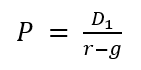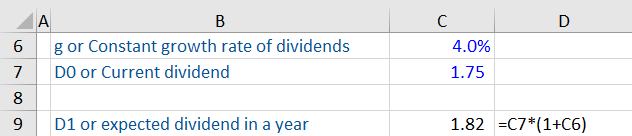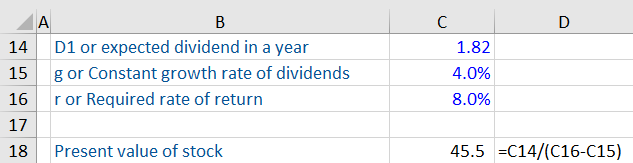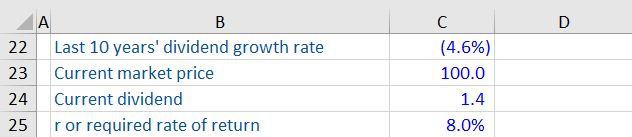## What is the Gordon Growth Model?

The Gordon Growth Model (GGM) is a popular model in finance and is commonly used to determine the value of a stock using future dividend payments. The model is named after Myron Gordon, an American economist, who popularized this model in the 1960s. In simple terms, the Gordon Growth Model calculates the present value of a future series of dividend payments. Here, the assumption is that future dividends will grow at a constant rate and will continue forever. Because of this assumption, this model can be applied only to companies with consistent growth in dividends.

## Key Learning Points

• The Gordon Growth Model or GGM is a valuation model used to calculate the value of a stock based on a future dividend
• The model relies on three variables: the forecasted dividend, required rate of return and the growth rate
• The model is limited by the assumption that dividends are assumed to grow at a constant rate (which is reality is very unlikely)
• Required rate of return is the return investors are willing to accept for losing their investment and cannot be lower than or equal to the dividend growth rate (G)
• A key assumption of the GGM model is that the company pays all of its equity free cash flows in dividends

## The Gordon Growth Model formula

Here is the formula for calculating the present value of a stock using the GGM:Where

P = Present value of stock

g = Constant growth rate at which dividends are expected to grow, in perpetuity

r = Required rate of return investors are willing to accept for risking their money on this stock

D1 = Expected dividend in the next year

The formula for the required rate of return is as follows:

r = Expected dividend payment/current share price + forecasted dividend growth rate

## The Gordon Growth Model Explained

Let us say a company’s dividends have been growing at a constant rate of 4%. Its current dividend is \$1.75, and its current stock price is \$30. Investors are expecting a return of 8%.

The first step is to calculate the expected dividend in the next year.Using the above information, you can calculate the present value of the stock as follows:The result indicates that the stock is worth \$45.5. The current market price is \$30, therefore, it is undervalued and is worth considering.

## Limitations of the Gordon Growth Model

Let us understand the limitations of this model with a real-world example. The dividend data of a global pharmaceutical company with a consistent history of dividend payments is shown below. As of Year 0, here is the information available for this stock:### Assumption of Consistent Growth in Dividends

The biggest limitation is that it requires constant growth in dividend payments. This is very unlikely in the real world where dividend growth fluctuates due to competitive, economic, and internal reasons. If you calculate the present value of this stock using the past 10 year’s dividend growth rate (which is negative,) you get the expected dividend in a year (1.34) that’s lower than the present dividend (1.40).

Assuming the investors are expecting a rate of return of 8%, the present value of the stock works out to \$10.6. That’s way off from the market value of the stock and thus is not reliable.

### The Required Rate of Return Cannot be Lower than or Equal to the Dividend Growth Rate

This is impossible as a share price cannot fall below zero. This principle is not a temporary phenomenon but applies to perpetuity. The required rate of return will always be higher than the dividend growth rate. Why? Because a company cannot grow permanently at a rate higher than the rate of return expected by investors. It is unrealistic because such a company would eventually grow to consume all of the world’s capital and take over the world.

### Isn’t a Company’s Value Based on Cash Flows Rather than Dividends?

A commonly asked question about GGM is why is it okay to value a company using dividends when other valuation techniques rely on earnings or cash flows? The key thing to remember is that even in GGM we are actually valuing cash flows here. A key assumption of the GGM model is that the company pays all of its equity free cash flows in dividends.

So even if the company is not paying dividends today (because it is reinvesting its profits to grow the business), those profits and cash flows will eventually be paid out as dividends once the company matures. However, this comes back to the limitation highlighted above. A company that is not paying any dividends now, but will start paying dividends when it matures, would have a non-constant dividend growth rate. And this will make it challenging to calculate the present value of its stock.